# Convective Heat Transfer: One Dimensional (Part - 5) Chemical Engineering Notes | EduRev

## Chemical Engineering : Convective Heat Transfer: One Dimensional (Part - 5) Chemical Engineering Notes | EduRev

The document Convective Heat Transfer: One Dimensional (Part - 5) Chemical Engineering Notes | EduRev is a part of the Chemical Engineering Course Heat Transfer for Engg..
All you need of Chemical Engineering at this link: Chemical Engineering

Lecture 13 - Convective Heat transfer: One dimensional, Heat Transfer

3.3.1 Analytical solution of the above cases
Case I:
The boundary conditions will be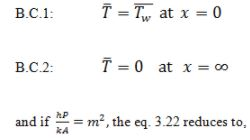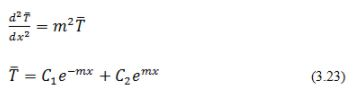Using boundary conditions, the solution of the equation 3.23 becomes,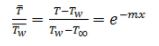All of the heat lost by the fin must have conducted from the base at x=0. Thus, we can compute the heat loss by the fin using the equation for temperature distribution,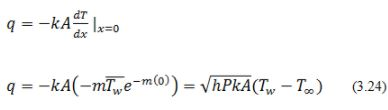Similarly, for Case – II, the boundary conditions are:The second boundary condition is a convective boundary condition which implies that the rate at which heat is conducted from inside the solid to the boundary is equal to the rate at which it is transported to the ambient fluid by convection.

The temperature profile is,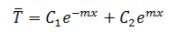or we can write,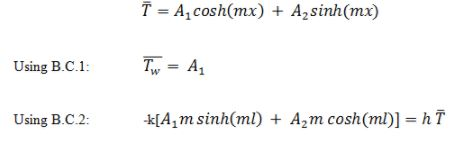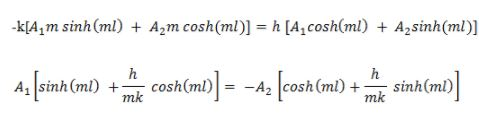thus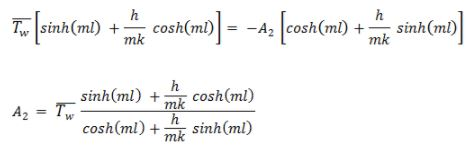Therefore, the boundary conditions led to the following solution to the eq.3.23.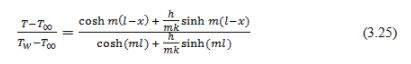Thus, the heat loss by the fin, using the equation for temperature distribution can be easily found out by the following equation,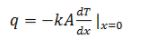(3.26)

In a similar fashion we can solve the case – III also. The boundary conditions are,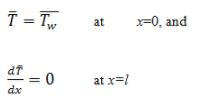Thus, on solving eq.3.23,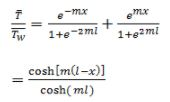Thus the heat loss by the fins, using the equation for temperature distribution,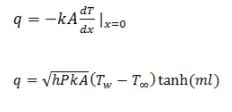(3.27)

It is to be noted that the general expression for the temperature gradient (eq.3.23) was developed by assuming the temperature gradient in the x-direction. It is really applicable with very less error, if the fin is sufficiently thin. However, for the practical fins the error introduced by this assumption is less than 1% only. Moreover, the practical fin calculation accuracy is limited by the uncertainties in the value of h. It is because the h value of the surrounding fluid is hardly uniform over the entire surface of the fin.

3.3.2 Fin efficiency
It was seen that the temperature of the fin decreases with distance x from the base of the body. Therefore, the driving force (temperature difference) also decreases with the length and hence the heat flux from the fin also decreases. It may also be visualized that if the thermal conductivity of the fin material is extremely high. Its thermal resistance will be negligibly small and the temperature will remain almost constant (Tw) throughout fin. In this condition the maximum heat transfer can be achieved and of-course it is an ideal condition. It is therefore, interesting and useful to calculate the efficiency of the fins.

The fin efficiency may be define as,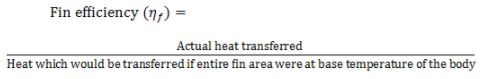Thus depending upon the condition, the actual heat transfer can be calculated as shown previously. As an example, for case – III (end of the fin is insulated), the rate of heat transfer was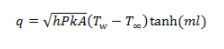(3.28)

The maximum heat would be transferred from the fin in an ideal condition in which the entire fin area was at Tw. In this ideal condition the heat transferred to the surrounding will be,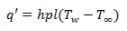(3.29)

Therefore, under such conditions, the efficiency of the fin will be;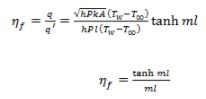(3.30)

where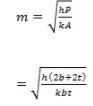If the fin is quite deep as compared to the thickness, the term 2b will be very large as compared to 2t, and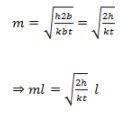(3.31)

The equation shows that the efficiency (from eq.3.30) of a fin which is insulated at the end can be easily calculated, which is the case-III discussed earlier. The efficiency for the other cases may also be evaluated in a similar fashion.

The above derivation is approximately same as of practical purposes, where the amount of heat loss from the exposed end is negligible.

It can be noted that the fin efficiency is maximum for the zero length of the fin (l = 0) or if there is no fin. Therefore, we should not expect to be able to maximize fin efficiency with respect to the fin length. However, the efficiency maximization should be done with respect to the quantity of the fin material keeping economic consideration in mind.

Sometimes the performance of the fin is compared on the basis of the rate of heat transfer with the fin and without the fin as shown,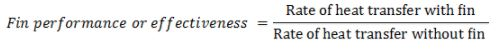Illustration 3.3.

A steel pipe having inner diameter as 78 mm and outer diameter as 89 mm has 10 external longitudinal rectangular fins of 1.5 mm thickness. Each of the fins extends 30 mm from the pipe. The thermal conductivity of the fin material is 50 W/m oC. The temperature of the pipe wall and the ambient are 160 oC, and 30 oC, respectively, whereas the surface heat transfer coefficient is 75 W/m2 oC. What is the percentage increase in the rate of heat transfer after the fin arrangement on the plane tube?

Solution 3.3

As the fins are rectangular, the perimeter of the fin, P = 2(b + t). The thickness (t) of the fin is quite small as compared to the width (b) of the fin. Thus, P = 2b as well as we may assume that there is no heat transfer from the tip of the fin. Under such condition we can treat it as case-III, where there was no heat transfer to the atmosphere due to insulated fin tip.
Using eq. 3.30,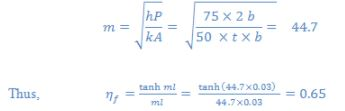As the pipe length is not given, we will work-out considering the length of the pipe as 1 m and henceforth the breadth of the fins should also be considered as 1 m. We have to consider the area of the fins in order to consider the heat dissipation from the fins. However, we may neglect the fin area at the y-z plane and x-y plane (refer fig. 3.8) as compared to the area of x-z plane.

The area of all the fins = (number of fins) (2 faces) (1) (0.03) = 0.6 m2

The maximum rate of heat transfer from the fins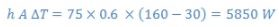Actual rate of heat transfer =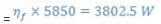The total rate of heat transfer from the finned tube will be the sum of actual rate of heat transfer from the fins and the rate of heat transfer from the bare pipe, the pipe portion which is not covered by the fins. Therefore, the remaining area will be calculated as follows,

The remaining area =  Total pipe area - base area covered by the 10 fins

Pipe are =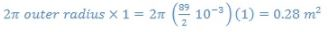Attached area of 10 fins = (10) (1) (0.0015) = 0.015 m2
The remaining area comes out to be (0.28 – 0.015) = 0.265 m2
The corresponding heat transfer = (75) (0.265) (160-30) = 2583.75 W
The total heat transfer from the finned tube = 3802.5 + 2583.75 = 6386.25 W
Rate of heat transfer from the tube if it does not have any fins = (75) (0.28) (160-30) = 2730 W
The percentage increase in the heat transfer =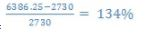Offer running on EduRev: Apply code STAYHOME200 to get INR 200 off on our premium plan EduRev Infinity!

## Heat Transfer for Engg.

46 videos|60 docs|59 tests

,

,

,

,

,

,

,

,

,

,

,

,

,

,

,

,

,

,

,

,

,

;